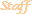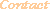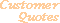Home Older Mathematica Items

Scientific Arts

# A Simple Analysis of Historical Sunspot Data

## Data Smoothing Using a Linear Filter

Here is a plot of the number of sunspots per month over a period of 2899 months. The data are contained in the file sunspots.dat and are read into the variable, SunspotData. (If the file sunspots.dat is not placed in a directory that is contained in Mathematica's \$Path variable, you can make it available by executing the functionwhere path is the path to the needed directory.)

``````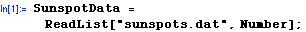``````

Let's set some plotting options globally for this session.

``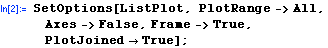``
``````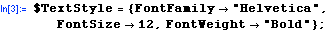``````

Here is quick visualization of the data.

````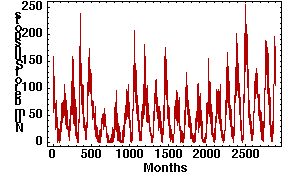This data can be "denoised" in a variety of ways. One very simple approach is to apply a "moving average" filter. This is most easily done using Mathematica's built in function ListConvolve which applies a linear filter kernel to an array of data (a list in this case). Here we do this with a kernel of length 12.

````
```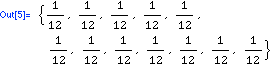```
``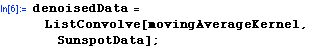``
``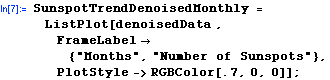``It is clear to the eye that there is an underlying periodicity to the data (indeed, this is the famous approximate 11 year sunspot cycle). Let's total the data on a yearly basis. This is a plot of the result: the month to month fluctuations in the first graph above are much greater than the year to year variations.

``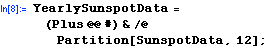``
``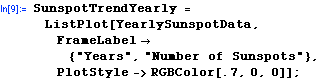``## Data Smoothing Using a Low Pass Filter

We can compute the frequency spectrum of this data through the use of Fourier which computes the discrete Fourier transform of a list of numbers. Here we show the absolute value of the resulting spectrum. The function Drop is used to remove the constant (zero frequency) component

````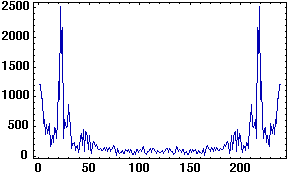The position of the absolute peak in this spectrum can be found using Position. Since we dropped the zero frequency term we add one to the result. The peak appears in two positions since the absolute value of the Fourier transform is symmetric about its center point.

````
```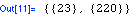```

If we remember that the discrete Fourier transform has the analytic representation (the actual algorithm used within Mathematica is of course a fast numerical algorithm which is not a direct application of this equation),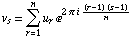we can see that the period corresponding to the peak can be computed from its position and the length of the data set. Thus, the period corresponding to this peak is estimated as (in years)

````
```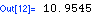```

The data also appears to have structure at low frequencies as well as a secondary peak near approximately 29 and another broad one near 46. These correspond to the respective periods,

``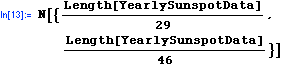``
``````

Let's low pass filter the Fourier transformed data to only take those frequencies corresponding to positions less than 50 (taking into account the zero frequency term).

``````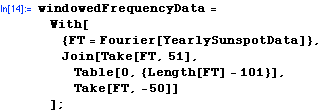``````

Now inverse Fourier transform the result and plot it.

````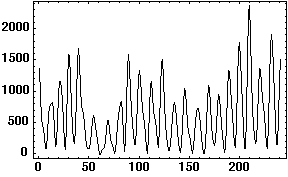Compare this to the original plot of the yearly data.

````This verifies our contention that the structure of this data is dominated by the low frequency information.

 For further information on our services send email to info@scientificarts.com . Contents of this web site Copyright © 1999-2011, Scientific Arts, LLC.
 s